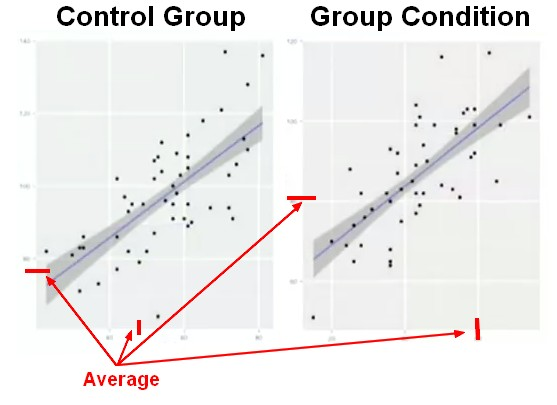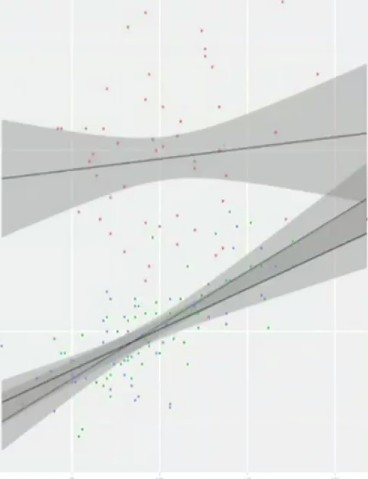# Statistics - Correlational Research

Correlation doesn't imply causation

A correlation research doesn't do any experimentation but uses survey, interviews, observations of behaviour as tools.

They looks like for pattern of correlation

A fundamental difference between experimental research and correlational research is that in correlational research there is no Independent variable (no groups)

Sometimes are they referred as study because no variable is manipulated (no independent variable)

A positive correlation (the slope of the line is going up)

A research that implies a quasi-independent variable is also a correlational research because the notion of randomness is not present.

Recommended PagesStatistics - (Interaction|Synergy) effect

In a multiple regression, is assumed that the effect on the target of increasing one unit of one predictor (is independent|has no influence) on the other predictor. If this is not the case, sharing a...Statistics - Factorial Anova

A factorial ANOVA is done when the independent variables are categorical. By adding a second independent variable, we are entering in factorial ANOVA. N Independent Variables (IVs). Variables that...Statistics - Mediator - Mediation (M)

Mediation is a different multivariate than moderation approach called mediation. mediation and moderation are very different kinds of analysis used to address very different types of questions. A mediator...Statistics - Moderator Variable (Z) - Moderation

A moderation analysis is a multiple regression analysis. The main reason to run a moderation analysis is to demonstrate how a third variable (Z) changes the correlation between two variables (X and Y)....Statistics - Research

Experimental research